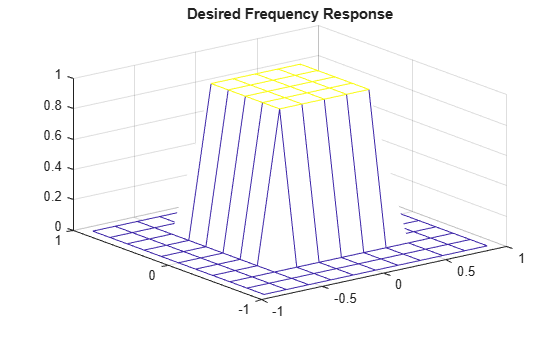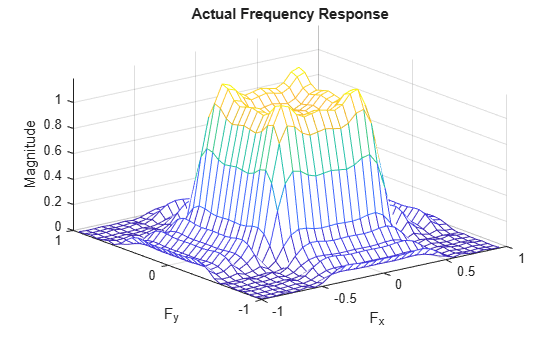## Design Linear Filters in the Frequency Domain

This topic describes functions that perform filtering in the frequency domain. For information about designing filters in the spatial domain, see What Is Image Filtering in the Spatial Domain?.

### Two-Dimensional Finite Impulse Response (FIR) Filters

The Image Processing Toolbox™ software supports one class of linear filter: the two-dimensional finite impulse response (FIR) filter. FIR filters have a finite extent to a single point, or impulse. All the Image Processing Toolbox filter design functions return FIR filters.

FIR filters have several characteristics that make them ideal for image processing in the MATLAB® environment:

• FIR filters are easy to represent as matrices of coefficients.

• Two-dimensional FIR filters are natural extensions of one-dimensional FIR filters.

• There are several well-known, reliable methods for FIR filter design.

• FIR filters are easy to implement.

• FIR filters can be designed to have linear phase, which helps prevent distortion.

Another class of filter, the infinite impulse response (IIR) filter, is not as suitable for image processing applications. It lacks the inherent stability and ease of design and implementation of the FIR filter. Therefore, this toolbox does not provide IIR filter support.

Note

Most of the design methods described in this section work by creating a two-dimensional filter from a one-dimensional filter or window created using Signal Processing Toolbox™ functions. Although this toolbox is not required, you might find it difficult to design filters if you do not have the Signal Processing Toolbox software.

### Create 2-D Filter Using Frequency Transformation of 1-D Filter

This example shows how to transform a one-dimensional FIR filter into a two-dimensional FIR filter using the `ftrans2` function. This function can be useful because it is easier to design a one-dimensional filter with particular characteristics than a corresponding two-dimensional filter. The frequency transformation method preserves most of the characteristics of the one-dimensional filter, particularly the transition bandwidth and ripple characteristics. The shape of the one-dimensional frequency response is clearly evident in the two-dimensional response.

This function uses a transformation matrix, a set of elements that defines the frequency transformation. This function's default transformation matrix produces filters with nearly circular symmetry. By defining your own transformation matrix, you can obtain different symmetries. (See Jae S. Lim, Two-Dimensional Signal and Image Processing, 1990, for details.)

Create 1-D FIR filter using the `firpm` function from the Signal Processing Toolbox.

```b = firpm(10,[0 0.4 0.6 1],[1 1 0 0]) ```
```b = Columns 1 through 9 0.0537 -0.0000 -0.0916 -0.0001 0.3131 0.4999 0.3131 -0.0001 -0.0916 Columns 10 through 11 -0.0000 0.0537```

Transform the 1-D filter to a 2-D filter.

```h = ftrans2(b); ```
```h = Columns 1 through 9 0.0001 0.0005 0.0024 0.0063 0.0110 0.0132 0.0110 0.0063 0.0024 0.0005 0.0031 0.0068 0.0042 -0.0074 -0.0147 -0.0074 0.0042 0.0068 0.0024 0.0068 -0.0001 -0.0191 -0.0251 -0.0213 -0.0251 -0.0191 -0.0001 0.0063 0.0042 -0.0191 -0.0172 0.0128 0.0259 0.0128 -0.0172 -0.0191 0.0110 -0.0074 -0.0251 0.0128 0.0924 0.1457 0.0924 0.0128 -0.0251 0.0132 -0.0147 -0.0213 0.0259 0.1457 0.2021 0.1457 0.0259 -0.0213 0.0110 -0.0074 -0.0251 0.0128 0.0924 0.1457 0.0924 0.0128 -0.0251 0.0063 0.0042 -0.0191 -0.0172 0.0128 0.0259 0.0128 -0.0172 -0.0191 0.0024 0.0068 -0.0001 -0.0191 -0.0251 -0.0213 -0.0251 -0.0191 -0.0001 0.0005 0.0031 0.0068 0.0042 -0.0074 -0.0147 -0.0074 0.0042 0.0068 0.0001 0.0005 0.0024 0.0063 0.0110 0.0132 0.0110 0.0063 0.0024 Columns 10 through 11 0.0005 0.0001 0.0031 0.0005 0.0068 0.0024 0.0042 0.0063 -0.0074 0.0110 -0.0147 0.0132 -0.0074 0.0110 0.0042 0.0063 0.0068 0.0024 0.0031 0.0005 0.0005 0.0001```

View the frequency response of the filters.

```[H,w] = freqz(b,1,64,'whole'); colormap(jet(64)) plot(w/pi-1,fftshift(abs(H))) figure, freqz2(h,[32 32]) ```

One-Dimensional Frequency ResponseCorresponding Two-Dimensional Frequency Response### Create Filter Using Frequency Sampling Method

This example shows how to create a 2-D filter based on a desired frequency response using the frequency sampling method.

Given a matrix of points that define the shape of the frequency response, the frequency sampling method creates a filter whose frequency response passes through those points. Frequency sampling places no constraints on the behavior of the frequency response between the given points. Usually, the response ripples in these areas. (Ripples are oscillations around a constant value. The frequency response of a practical filter often has ripples where the frequency response of an ideal filter is flat.)

Define and display the target 2-D frequency response of an 11-by-11 filter.

```Hd = zeros(11,11); Hd(4:8,4:8) = 1; [f1,f2] = freqspace(11,"meshgrid"); mesh(f1,f2,Hd) colormap(jet(64)) title("Desired Frequency Response")```Create the filter based on the target frequency response using the `fsamp2` function. `fsamp2` returns the filter `h` with a frequency response that passes through the points in the input matrix `Hd`

`h = fsamp2(Hd);`

Plot the frequency response of the filter.

```freqz2(h,[32 32]) title("Actual Frequency Response")```Notice the ripples in the actual frequency response compared to the desired frequency response. These ripples are a fundamental problem with the frequency sampling design method. They occur wherever there are sharp transitions in the desired response.

You can reduce the spatial extent of the ripples by using a larger filter. However, a larger filter does not reduce the height of the ripples, and requires more computation time for filtering. To achieve a smoother approximation to the desired frequency response, consider using the frequency transformation method or the windowing method.

### Windowing Method

The windowing method involves multiplying the ideal impulse response with a window function to generate a corresponding filter, which tapers the ideal impulse response. Like the frequency sampling method, the windowing method produces a filter whose frequency response approximates a desired frequency response. The windowing method, however, tends to produce better results than the frequency sampling method.

The toolbox provides two functions for window-based filter design, `fwind1` and `fwind2`. `fwind1` designs a two-dimensional filter by using a two-dimensional window that it creates from one or two one-dimensional windows that you specify. `fwind2` designs a two-dimensional filter by using a specified two-dimensional window directly.

`fwind1` supports two different methods for making the two-dimensional windows it uses:

• Transforming a single one-dimensional window to create a two-dimensional window that is nearly circularly symmetric, by using a process similar to rotation

• Creating a rectangular, separable window from two one-dimensional windows, by computing their outer product

The example below uses `fwind1` to create an 11-by-11 filter from the desired frequency response `Hd`. The example uses the Signal Processing Toolbox `hamming` function to create a one-dimensional window, which `fwind1` then extends to a two-dimensional window.

```Hd = zeros(11,11); Hd(4:8,4:8) = 1; [f1,f2] = freqspace(11,'meshgrid'); mesh(f1,f2,Hd), axis([-1 1 -1 1 0 1.2]), colormap(jet(64)) h = fwind1(Hd,hamming(11)); figure, freqz2(h,[32 32]), axis([-1 1 -1 1 0 1.2]) ```

Desired Two-Dimensional Frequency Response (left) and Actual Two-Dimensional Frequency Response (right)### Creating the Desired Frequency Response Matrix

The filter design functions `fsamp2`, `fwind1`, and `fwind2` all create filters based on a desired frequency response magnitude matrix. Frequency response is a mathematical function describing the gain of a filter in response to different input frequencies.

You can create an appropriate desired frequency response matrix using the `freqspace` function. `freqspace` returns correct, evenly spaced frequency values for any size response. If you create a desired frequency response matrix using frequency points other than those returned by `freqspace`, you might get unexpected results, such as nonlinear phase.

For example, to create a circular ideal low-pass frequency response with cutoff at 0.5, use

```[f1,f2] = freqspace(25,'meshgrid'); Hd = zeros(25,25); d = sqrt(f1.^2 + f2.^2) < 0.5; Hd(d) = 1; mesh(f1,f2,Hd) ```

Ideal Circular Low-pass Frequency ResponseNote that for this frequency response, the filters produced by `fsamp2`, `fwind1`, and `fwind2` are real. This result is desirable for most image processing applications. To achieve this in general, the desired frequency response should be symmetric about the frequency origin (`f1` `=` `0`, `f2` `=` `0`).

### Computing the Frequency Response of a Filter

The `freqz2` function computes the frequency response for a two-dimensional filter. With no output arguments, `freqz2` creates a mesh plot of the frequency response. For example, consider this FIR filter,

```h =[0.1667 0.6667 0.1667 0.6667 -3.3333 0.6667 0.1667 0.6667 0.1667];```

This command computes and displays the 64-by-64 point frequency response of `h`.

`freqz2(h)`

Frequency Response of a Two-Dimensional FilterTo obtain the frequency response matrix `H` and the frequency point vectors `f1` and `f2`, use output arguments

`[H,f1,f2] = freqz2(h);`

`freqz2` normalizes the frequencies `f1` and `f2` so that the value 1.0 corresponds to half the sampling frequency, or π radians.

For a simple `m`-by-`n` response, as shown above, `freqz2` uses the two-dimensional fast Fourier transform function `fft2`. You can also specify vectors of arbitrary frequency points, but in this case `freqz2` uses a slower algorithm.

See Fourier Transform for more information about the fast Fourier transform and its application to linear filtering and filter design.